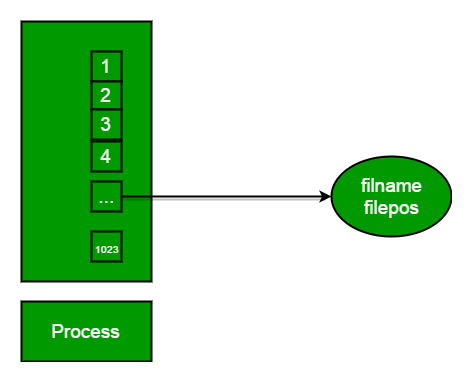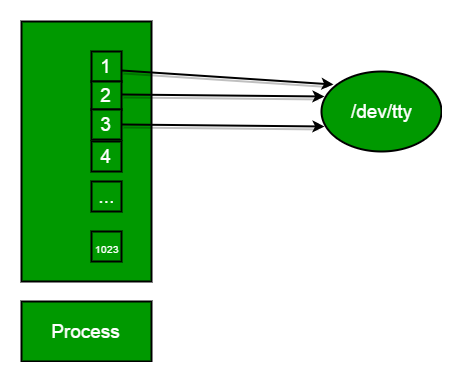# Input-output system calls in C | Create, Open, Close, Read, Write

Important Terminology

What is the File Descripter??
File descriptor is integer that uniquely identifies an open file of the process.

File Descriptor table: File descriptor table is the collection of integer array indices that are file descriptors in which elements are pointers to file table entries. One unique file descriptors table is provided in operating system for each process.

File Table Entry: File table entries is a structure In-memory surrogate for an open file, which is created when process request to opens file and these entries maintains file position.Standard File Descriptors: When any process starts, then that process file descriptors table’s fd(file descriptor) 0, 1, 2 open automatically, (By default) each of these 3 fd references file table entry for a file named /dev/tty

/dev/tty: In-memory surrogate for the terminal
Terminal: Combination keyboard/video screenRead from stdin => read from fd 0 : Whenever we write any character from keyboard, it read from stdin through fd 0 and save to file named /dev/tty.
Write to stdout => write to fd 1 : Whenever we see any output to the video screen, it’s from the file named /dev/tty and written to stdout in screen through fd 1.
Write to stderr => write to fd 2 : We see any error to the video screen, it is also from that file write to stderr in screen through fd 2.

I/O System calls

Basically there are total 5 types of I/O system calls:

1. Create: Used to Create a new empty file.
```Syntax in C language:
int creat(char *filename, mode_t mode)
```
Parameter :

• filename : name of the file which you want to create
• mode : indicates permissions of new file.
Returns :

• return first unused file descriptor (generally 3 when first creat use in process beacuse 0, 1, 2 fd are reserved)
• return -1 when error
How it work in OS

• Create new empty file on disk
• Create file table entry
• Set first unused file descriptor to point to file table entry
• Return file descriptor used, -1 upon failure
2. open: Used to Open the file for reading, writing or both.
```Syntax in C language
#include<sys/types.h>
#includ<sys/stat.h>
#include <fcntl.h>
int open (const char* Path, int flags [, int mode ]);
```

Parameters

• Path : path to file which you want to use
• use absolute path begin with “/”, when you are not work in same directory of file.
• Use relative path which is only file name with extension, when you are work in same directory of file.
• flags : How you like to use
• O_RDONLY: read only, O_WRONLY: write only, O_RDWR: read and write, O_CREAT: create file if it doesn’t exist, O_EXCL: prevent creation if it already exists
How it works in OS

• Find existing file on disk
• Create file table entry
• Set first unused file descriptor to point to file table entry
• Return file descriptor used, -1 upon failure

 `// C program to illustrate ` `// open system call ` `#include ` `#include ` `#include ` `extern` `int` `errno``; ` `int` `main() ` `{      ` `    ``// if file does not have in directory  ` `    ``// then file foo.txt is created. ` `    ``int` `fd = open(``"foo.txt"``, O_RDONLY | O_CREAT);  ` `     `  `    ``printf``(``"fd = %d/n"``, fd); ` `     `  `    ``if` `(fd ==-1) ` `    ``{ ` `        ``// print which type of error have in a code ` `        ``printf``(``"Error Number % d\n"``, ``errno``);  ` `         `  `        ``// print program detail "Success or failure" ` `        ``perror``(``"Program"``);                  ` `    ``} ` `    ``return` `0; ` `} `

Output:

```fd = 3
```
3. close: Tells the operating system you are done with a file descriptor and Close the file which pointed by fd.
```Syntax in C language
#include <fcntl.h>
int close(int fd);
```
Parameter

• fd :file descriptor
Return

• 0 on success.
• -1 on error.
How it works in the OS

• Destroy file table entry referenced by element fd of file descriptor table
– As long as no other process is pointing to it!
• Set element fd of file descriptor table to NULL

 `// C program to illustrate close system Call ` `#include ` `#include ` `int` `main() ` `{ ` `    ``int` `fd1 = open(``"foo.txt"``, O_RDONLY); ` `    ``if` `(fd1 < 0)  ` `    ``{ ` `        ``perror``(``"c1"``); ` `        ``exit``(1); ` `    ``} ` `    ``printf``(``"opened the fd = % d\n"``, fd1); ` `     `  `    ``// Using close system Call ` `    ``if` `(close(fd1) < 0)  ` `    ``{ ` `        ``perror``(``"c1"``); ` `        ``exit``(1); ` `    ``}  ` `    ``printf``(``"closed the fd.\n"``); ` `} `

Output:

```opened the fd = 3
closed the fd.
```

 `// C program to illustrate close system Call ` `#include ` `#include ` `int` `main() ` `{ ` `    ``// assume that foo.txt is already created ` `    ``int` `fd1 = open(``"foo.txt"``, O_RDONLY, 0);  ` `    ``close(fd1); ` `     `  `    ``// assume that baz.tzt is already created ` `    ``int` `fd2 = open(``"baz.txt"``, O_RDONLY, 0);  ` `     `  `    ``printf``(``"fd2 = % d\n"``, fd2); ` `    ``exit``(0); ` `}  `

Output:

```fd2 = 3
```

Here, In this code first open() returns 3 because when main process created, then fd 0, 1, 2 are already taken by stdin, stdout and stderr. So first unused file descriptor is 3 in file descriptor table. After that in close() system call is free it this 3 file descriptor and then after set 3 file descriptor as null. So when we called second open(), then first unused fd is also 3. So, output of this program is 3.

4. read: From the file indicated by the file descriptor fd, the read() function reads cnt bytes of input into the memory area indicated by buf. A successful read() updates the access time for the file.
```Syntax in C language
size_t read (int fd, void* buf, size_t cnt);
```
Parameters

• fd: file descripter
• buf: buffer to read data from
• cnt: length of buffer
Returns: How many bytes were actually read

• return Number of bytes read on success
• return 0 on reaching end of file
• return -1 on error
• return -1 on signal interrupt
Important points

• buf needs to point to a valid memory location with length not smaller than the specified size because of overflow.
• fd should be a valid file descriptor returned from open() to perform read operation because if fd is NULL then read should generate error.
• cnt is the requested number of bytes read, while the return value is the actual number of bytes read. Also, some times read system call should read less bytes than cnt.

 `// C program to illustrate ` `// read system Call ` `#include ` `#include ` `int` `main() ` `{ ` `  ``int` `fd, sz; ` `  ``char` `*c = (``char` `*) ``calloc``(100, ``sizeof``(``char``)); ` ` `  `  ``fd = open(``"foo.txt"``, O_RDONLY); ` `  ``if` `(fd < 0) { ``perror``(``"r1"``); ``exit``(1); } ` ` `  `  ``sz = read(fd, c, 10); ` `  ``printf``(``"called read(% d, c, 10).  returned that"` `         ``" %d bytes  were read.\n"``, fd, sz); ` `  ``c[sz] = ``'\0'``; ` `  ``printf``(``"Those bytes are as follows: % s\n"``, c); ` `} `

Output:

```called read(3, c, 10).  returned that 10 bytes  were read.
Those bytes are as follows: 0 0 0 foo.
```

Suppose that foobar.txt consists of the 6 ASCII characters “foobar”. Then what is the output of the following program?

 `// C program to illustrate ` `// read system Call ` `#include ` `#include ` ` `  `int` `main() ` `{ ` `    ``char` `c; ` `    ``int` `fd1 = Open(``"foobar.txt"``, O_RDONLY, 0); ` `    ``int` `fd2 = Open(``"foobar.txt"``, O_RDONLY, 0); ` `    ``Read(fd1, &c, 1); ` `    ``Read(fd2, &c, 1); ` `    ``printf``(``"c = % c\n"``, c); ` `    ``exit``(0); ` `} `

Output:

```c = f
```

The descriptors fd1 and fd2 each have their own open file table entry, so each descriptor has its own file position for foobar.txt. Thus, the read from fd2 reads the first byte of foobar.txt, and the output is c = f, not c = o.

5. write: Writes cnt bytes from buf to the file or socket associated with fd. cnt should not be greater than INT_MAX (defined in the limits.h header file). If cnt is zero, write() simply returns 0 without attempting any other action.
```#include <fcntl.h>
size_t write (int fd, void* buf, size_t cnt);
```
Parameters

• fd: file descripter
• buf: buffer to write data to
• cnt: length of buffer
Returns: How many bytes were actually written

• return Number of bytes written on success
• return 0 on reaching end of file
• return -1 on error
• return -1 on signal interrupt
Important points

• The file needs to be opened for write operations
• buf needs to be at least as long as specified by cnt because if buf size less than the cnt then buf will lead to the overflow condition.
• cnt is the requested number of bytes to write, while the return value is the actual number of bytes written. This happens when fd have a less number of bytes to write than cnt.
• If write() is interrupted by a signal, the effect is one of the following:
-If write() has not written any data yet, it returns -1 and sets errno to EINTR.
-If write() has successfully written some data, it returns the number of bytes it wrote before it was interrupted.

 `// C program to illustrate ` `// write system Call ` `#include ` `#include ` `main() ` `{ ` `  ``int` `sz; ` ` `  `  ``int` `fd = open(``"foo.txt"``, O_WRONLY | O_CREAT | O_TRUNC, 0644); ` `  ``if` `(fd < 0) ` `  ``{ ` `     ``perror``(``"r1"``); ` `     ``exit``(1); ` `  ``} ` ` `  `  ``sz = write(fd, ``"hello geeks\n"``, ``strlen``(``"hello geeks\n"``)); ` ` `  `  ``printf``(``"called write(% d, \"hello geeks\\n\", %d)."` `    ``" It returned %d\n"``, fd, ``strlen``(``"hello geeks\n"``), sz); ` ` `  `  ``close(fd); ` `} `

Output:

```called write(3, "hello geeks\n", 12).  it returned 11
```

Here, when you see in the file foo.txt after running the code, you get a “hello geeks“. If foo.txt file already have some content in it then write system call overwrite the content and all previous content are deleted and only “hello geeks” content will have in the file.

Print “hello world” from the program without use any printf or cout function.

 `// C program to illustrate ` `// I/O system Calls ` `#include ` `#include ` `#include ` `#include ` ` `  `int` `main (``void``) ` `{ ` `    ``int` `fd; ` `    ``char` `buf1 = ``"hello world"``; ` `    ``char` `buf2; ` ` `  `    ``// assume foobar.txt is already created ` `    ``fd = open(``"foobar.txt"``, O_RDWR);         ` `    ``fd = open(``"foobar.txt"``, O_RDWR); ` `     `  `    ``write(fd, buf1, ``strlen``(buf1));          ` `    ``write(1, buf2, read(fd, buf2, 12)); ` ` `  `    ``close(fd); ` `    ``close(fd); ` ` `  `    ``return` `0; ` `} `

Output:

```hello world
```

In this code, buf1 array’s string “hello world” is first write in to stdin fd then after that this string write into stdin to buf2 array. After that write into buf2 array to the stdout and print output “hello world“.

My Personal Notes arrow_drop_up

Improved By : ritwikshanker

Article Tags :
Practice Tags :

12

Please write to us at contribute@geeksforgeeks.org to report any issue with the above content.